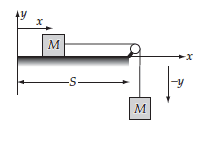# Lagrange equations: Two blocks and a string

Zamarripa
Homework Statement:
Two blocks, each of mass M, are connected by an extensionless, uniform string of length l. One block is placed on a smooth horizontal surface, and the other block hangs over the side, the string passing over a frictionless pulley. Describe the motion of the system in two cases, first when the mass of the string is negligible, and second when the string has a mass m.
Relevant Equations:
$$T_{string}=\frac{1}{2}m\dot{y}$$
I've problems understanding why the kinetic energy of the string is only$$T_{string}=\frac{1}{2}m\dot{y}$$

Why the contribution of the string in the horizontal line isn't considered?

Homework Helper
Gold Member
2022 Award
What's the formula for KE?

Zamarripa
What's the formula for KE?
$$T=\frac{1}{2}mv^2$$

Homework Helper
Gold Member
2022 Award
$$T=\frac{1}{2}mv^2$$
Which is not what you're using?

Zamarripa
Yes, but what about the contribution on the horizontal?

Homework Helper
Gold Member
2022 Award
Yes, but what about the contribution on the horizontal?

What contribution from the horizontal?

The problem is that you have ##\frac 1 2 m \dot y## instead of ##\frac 1 2 m \dot y^2##.

Zamarripa
What contribution from the horizontal?

The problem is that you have ##\frac 1 2 m \dot y## instead of ##\frac 1 2 m \dot y^2##.
The string at the horizontal is also moving

Homework Helper
Gold Member
2022 Award
The string at the horizontal is also moving
So, what do you think the KE of the string should be?

Zamarripa
So, what do you think the KE of the string should be?
$$\frac{1}{2}m\frac{(s-x)}{l}\dot{x}$$

where ##m\frac{(s-x)}{l}## is the mass of the string in the horizontal

Homework Helper
Gold Member
2022 Award
$$\frac{1}{2}m\frac{(s-x)}{l}\dot{x}$$

where ##m\frac{(s-x)}{l}## is the mass of the string in the horizontal

1) The whole string is moving, so you need the whole mass of the string: ##m##.

2) ##\dot x = \dot y##, as the string is inextensible.

3) You need to use ##v^2 = \dot x^2 = \dot y^2## for KE. You can't use ##\dot x## or ##\dot y##.

•vanhees71